# R Tutorial

  Copyright (c) 2013-2020 Aurelien Ginolhac, UL HPC Team  <hpc-sysadmins@uni.lu>Through this tutorial you will learn how to use R from your local machine or from one of the UL HPC platform clusters. Then, we will see how to organize and group data. Finally we will illustrate how R can benefit from multicore and cluster parallelization.

Warning: this tutorial does not focus on the learning of R language but aims at showing you nice start-up tips. If you’re also looking for a good tutorial on R’s data structures you can take a look at: Hadley Wickham’s page. Another bookdown’s book is available for free: R for Data Science by Garrett Grolemund & Hadley Wickham

## Pre-requisites

Ensure you are able to connect to the UL HPC clusters

you MUST work on a computing node

# /!\ FOR ALL YOUR COMPILING BUSINESS, ENSURE YOU WORK ON A COMPUTING NODE
(access-iris)$> si --time=0:30:0 -N 1 --ntasks-per-node=1 --cpus-per-task=2 --mem-per-cpu 4096  ### Optional: On your local machine First of all, let’s install R. You will find releases for various distributions available at CRAN Archive. Once installed, to use R interactive session interface, simply open a terminal and type: jdoe@localhost:~$ R


You will also find handy to use the R-Studio graphical IDE. R-Studio embeds a R shell where you can call R functions as in the interactive session interface. Thus you can use whether R interactive shell or R-Studio embedded shell.

### Installing R Packages

To install libraries you can use the install.packages() function. e.g

install.packages("tidyverse")

This will install the core packages of the tidyverse, including ggplot2 and dplyr.

Note: on the first run, R might ask you various questions during the installation. e.g. selecting a CRAN mirror to use for downloading packages. Select a mirror close to your location. For other questions, using default values is ok.

Now, to load packages with a library() call:

library(ggplot2)

## Beginner session – dataSaurus

in R or Rstudio do install.packages("tidyverse") (takes some time)

instructions: in this tutorial

## Comparing methods for aggregating data – diamonds

Let’s say we are working with the full diamonds dataset (supplied by the ggplot2 package) and we want to compute the average price for a given diamond cut.

This dataset is not small:

dim(diamonds)

##  53940    10


before computing the average price per cut, we should explore the dataset. We won’t use the ggplot’s function geom_point() as there are too many dots. However, binning the data is fast and still provide an idea of where the majority of dots are with a chosen lost of precision (number of bins, set to 30 by default).

From previous plots, we know the relationship between carat and price is linear in the log-space. All those computation can be done by ggplot2

Of note: you need to install the package hexbin

ggplot(diamonds) +
# bin data into 30 sets
geom_hex(aes(x = carat, y = price), bins = 30) +
# split per cut
facet_wrap(~ cut) +
# log transform both axes
scale_x_log10() +
scale_y_log10() +
# add logsticks to bottom and left
annotation_logticks(side = "bl") +
# viridis is so much better than the default blue gradient
scale_fill_viridis_c() +
theme_minimal(14)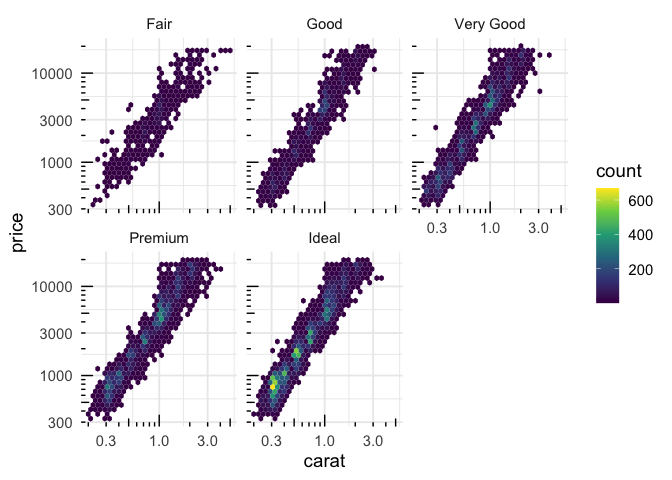#ggsave(file = "diamonds_plot.pdf", last_plot(), width = 8, height = 4)


### Speed comparison of different tools for aggregating data

We could do a for loop to aggregate the data per cuts and manually compute the average price, but in R loops are generally a bad idea (if you avoid growing vectors there are fine) and:It is better to concentrate on actions and not on programming, so the looping can be efficiently done internally.

Thus instead of looping around the dataset, we will use a function from the dplyr package part of the tidyverse idiom

#### dplyr from the tidyverse

First of all, we chain the commands using the pipe %>%. That avoids many parenthesis and keep the natural reading from left to right. Of note, the pipe |> will come in R base with the next version, 4.1.

The first parameter will be the dataset, the second will be the column of the dataset we want to group the results on, third parameter will be the call to the summarise() function that will enable to aggregate data on the cut column.

library(dplyr)
diamonds %>%
group_by(cut) %>%
summarise(avg_price = mean(price))

## summarise() ungrouping output (override with .groups argument)

## # A tibble: 5 x 2
##   cut       avg_price
##   <ord>         <dbl>
## 1 Fair          4359.
## 2 Good          3929.
## 3 Very Good     3982.
## 5 Ideal         3458.


Note: summarise() from the dplyr package is similar to aggregate() from base package, dplyr functions simply provide a more consistent naming convention together with better performance

#### aggregate from base

aggregate(price ~ cut,
FUN = mean,
data = diamonds)

##         cut    price
## 1      Fair 4358.758
## 2      Good 3928.864
## 3 Very Good 3981.760
## 5     Ideal 3457.542


### lapply from base

In the previous example we used aggregate for the aggregation, we could also have used lapply (but in a more complex way):

as.data.frame(cbind(cut = as.character(unique(diamonds$cut)), avg_price = lapply(unique(diamonds$cut),
function(x) {
mean(diamonds$price[which(diamonds$cut == x)])
})))

##         cut avg_price
## 1     Ideal  3457.542
## 3      Good  3928.864
## 4 Very Good   3981.76
## 5      Fair  4358.758


#### data.table

data.table is a package without dependencies that is super fast. Although the syntax is harder to learn compared to dplyr, if speed is your concern, it is your go-to package. See this long thread at stackoverflow for a comparison of both tools.

# install.package("data.table")
suppressPackageStartupMessages(library(data.table))
DT <- data.table(diamonds)
DT[, mean(price), by = cut]

##          cut       V1
## 1:     Ideal 3457.542
## 3:      Good 3928.864
## 4: Very Good 3981.760
## 5:      Fair 4358.758


#### benchmarks

So, we want to know which one of the two versions is the most efficient, for that purpose, the package bench is handy.

install.packages("bench")


The mark() function performs x times the calls of several expressions, grabbing its performance (time and ressources used)

library(bench)
set.seed(123)
m <- bench::mark(LAPPLY    = as.data.frame(cbind(cut = as.character(unique(diamonds$cut)), price = lapply(unique(diamonds$cut), function(x) mean(diamonds$price[which(diamonds$cut == x)])))),
AGGREGATE = aggregate(price ~ cut, FUN = mean, data = diamonds),
DPLYR     = group_by(diamonds, cut) %>% summarise(price = mean(price)),
DATATABLE = DT[, list(price = mean(price)), by = cut],
iterations = 100, check = FALSE)

• makes comparison easier to read using relative values. 1 for the fastest.
summary(m, relative = TRUE)

## # A tibble: 4 x 6
##   expression   min median itr/sec mem_alloc gc/sec
##   <bch:expr> <dbl>  <dbl>     <dbl>     <dbl>    <dbl>
## 1 LAPPLY      5.51   5.46      3.55      5.99     2.22
## 2 AGGREGATE  21.6   23.8       1         9.13     1.58
## 3 DPLYR       2.67   2.84      6.47      1        1
## 4 DATATABLE   1      1        19.4       1.23     1.81

• plotting the benchmark

You can use ggplot2 via the autoplot() function

autoplot(m)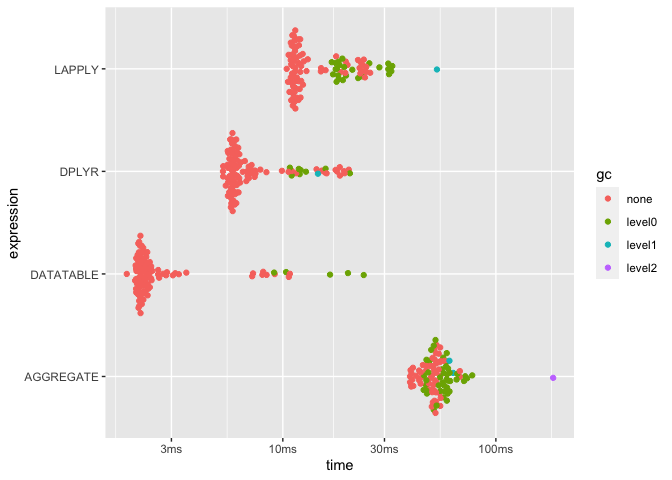## Parallel computing using HPC

R is already available on iris clusters as a module. The current production version is 3.6.0. The first step is the reservation of a resource. Connect to your favorite cluster frontend (here: iris). We assume you have already configured your .ssh/config.

#### iris

jdoe@localhost:~$ssh iris-cluster  Once connected to the user frontend, book 4 cores for half an hour [jdoe@access2 ~]$ si --time=0:30:0 -N 1 --ntasks-per-node=1 --cpus-per-task=4 --mem-per-cpu 4096


When the job is running and you are connected load R module (so version 3.6.0).

[jdoe@access2 ~]$module load lang/R  Now you should be able to invoke R and see something like this: [jdoe@iris-081 ~]$  R

R version 3.6.0 (2019-04-26) -- "Planting of a Tree"
Copyright (C) 2019 The R Foundation for Statistical Computing
Platform: x86_64-pc-linux-gnu (64-bit)

[...]
Type 'q()' to quit R.
>


### mandatory packages

The core package we are going to use is future by Henrik Bengtsson.

The main idea is to run expressions asynchronously. Future expressions are run according to plan defined by the user.

for the last section, you need to install the following packages, future, furrr and Rstne

module load lang/R/3.6.0-intel-2019a-bare


you will a warning because it hasn’t been fully tested yet.

After entering R using the R command, install both packages. Don’t forget to use multi-threading for the compilations.

install.packages(c("future", "furrr", "purrr", "dplyr", "dbscan", "tictoc", "Rtsne"),
repos = "https://cran.rstudio.com", Ncpus = 4)


quit by typing quit() and n do not save workspace image (actually, this should be your default).

The furrr package by David Vaughan is a nice wrapper around future and purrr, the functional programming idiom of the tidyverse.

### toy example

Run a dumb loop on 3 times 2 that wait for those values

• first sequentially
library(furrr)

## Loading required package: future

plan(sequential)
tictoc::tic()
nothingness <- future_map(c(2, 2, 2), ~ Sys.sleep(.x), .progress = TRUE)
tictoc::toc()

## 6.045 sec elapsed

• second in parallel
plan(multisession)
tictoc::tic()
nothingness <- future_map(c(2, 2, 2), ~ Sys.sleep(.x), .progress = TRUE)
tictoc::toc()

## 2.413 sec elapsed


fetch env variables in R

as.integer(Sys.getenv("SLURM_CPUS_PER_TASK"))

### t-SNE example

Now, let’s try with a real example. Using some data supplied as a R object.

Create both the R and bash script in a folder (likely your scratch partition).

#### R script

library(dplyr)
library(purrr)
library(furrr)
library(Rtsne)

# check the path of pkgs
.libPaths()
# see how many cores are going to be used
availableCores()
# create a partial function with filled arguments
short_tsne <- purrr::partial(Rtsne, X = tSNEdata, pca_center = TRUE,
theta = 0.5, pca_scale = TRUE, verbose = TRUE, max_iter = 300)

plan(multiprocess)

tictoc::tic(msg = "tsne")
tsne_future <- tibble(perplexities = seq(10, 110, by = 5)) %>%
# quietly captures outputs
mutate(model = future_map(perplexities, ~quietly(short_tsne)(perplexity = .x), .progress = FALSE))
tictoc::toc()

tictoc::tic("finding clusters")
res_tib <- mutate(tsne_future,
# unlist and extract the 2D matrix
Y = map(model, pluck, "result", "Y"),
# convert to a dataframe
Y_df = map(Y, as.data.frame),
# for clustering, parallelise since expensive step
cluster = future_map(Y_df, dbscan::hdbscan, minPts = 200),
# extract from hdbscan object only the cluster info
c = map(cluster, pluck, 1),
# iterate though the 2D coordinates and cluster info to merge them
tsne = map2(Y_df, c, ~ bind_cols(.x, tibble(c = .y))),
# extract the output of tsne runs if needed to be parsed
output = map_chr(model, pluck, "output"))
tictoc::toc()

saveRDS(res_tib, "tsne_future.rds")


save as file named tsne.R

#### launcher for 10 minutes on one full node (28 cores)

#!/bin/bash -l
#SBATCH -J tsne_hpc
#SBATCH -N 1
#SBATCH -c 28
#SBATCH --time=0-00:10:00
#SBATCH -p batch
#SBATCH --qos=normal

echo "== Starting run at $(date)" echo "== Job ID:${SLURM_JOBID}"
echo "== Node list: ${SLURM_NODELIST}" echo "== Submit dir. :${SLURM_SUBMIT_DIR}"

# use version 3.6.0 and load the GNU toolchain

# prevent sub-spawn, 28 cores -> 28 processes

Rscript tsne.R > job_${SLURM_JOB_NAME}.out 2>&1  save as file named launcher.sh. It requests a full node of 28 cores #### Run the job on the iris frontend, in the folder where both the tsne.R and launcher.sh files are located, type the slurm command: sbatch launcher.sh  you can monitor the queue line with: squeue -l -u$USER


When your passive job has started, you can connect to the assigned node using the sjoin command on the frontend by Valentin Plugaru, use to autocomplete the correct job and node ids.

Once logged in, you can check that the job is indeed run using the 28 cores as the output of htop below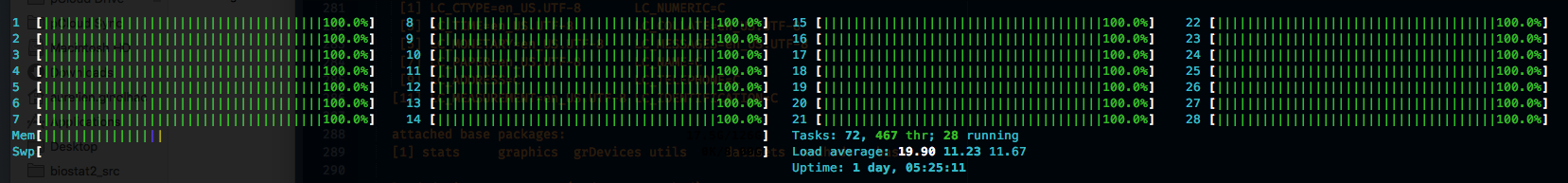and that they are dully full processes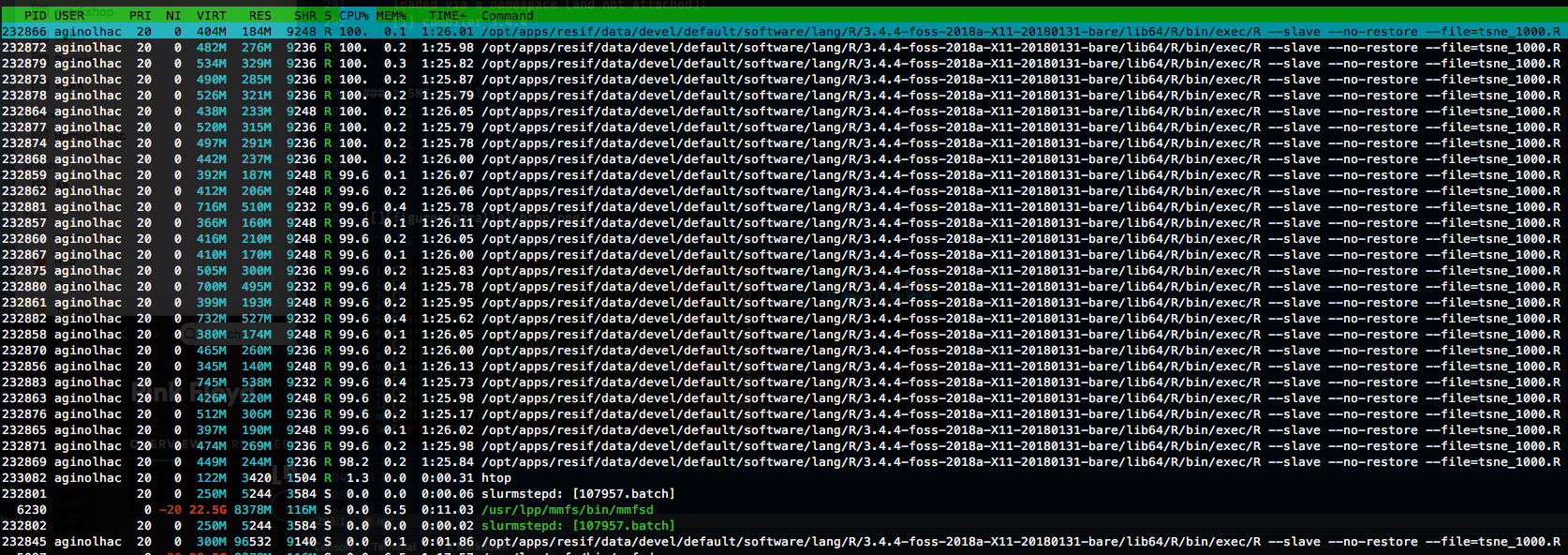furrr allows to simply change your map() functions to future_map() to run in parallel. future takes in charge all the spawning and fetching of the processes.

it should give the following timing, looking at the job_tsne_hpc.out file:

tsne: 16.103 sec elapsed
finding clusters: 2.395 sec elapsed

##### trying the sequential version
• remove the future calls

sed 's/future_map/map/' tsne.R > tsne_nofuture.R

sed 's/tsne.R/tsne_nofuture.R/' launcher.sh > launcher_nofuture.sh

• submit the job

sbatch launcher_nofuture.sh

• new timing, same job_tsne_hpc.out file:
tsne: 214.913 sec elapsed
finding clusters: 28.043 sec elapsed


#### Conclusion

see below the benefit of using more cores on the elapsed time for computationally intensive tasks such as t-SNE (code in furrr_benchmark.Rmd)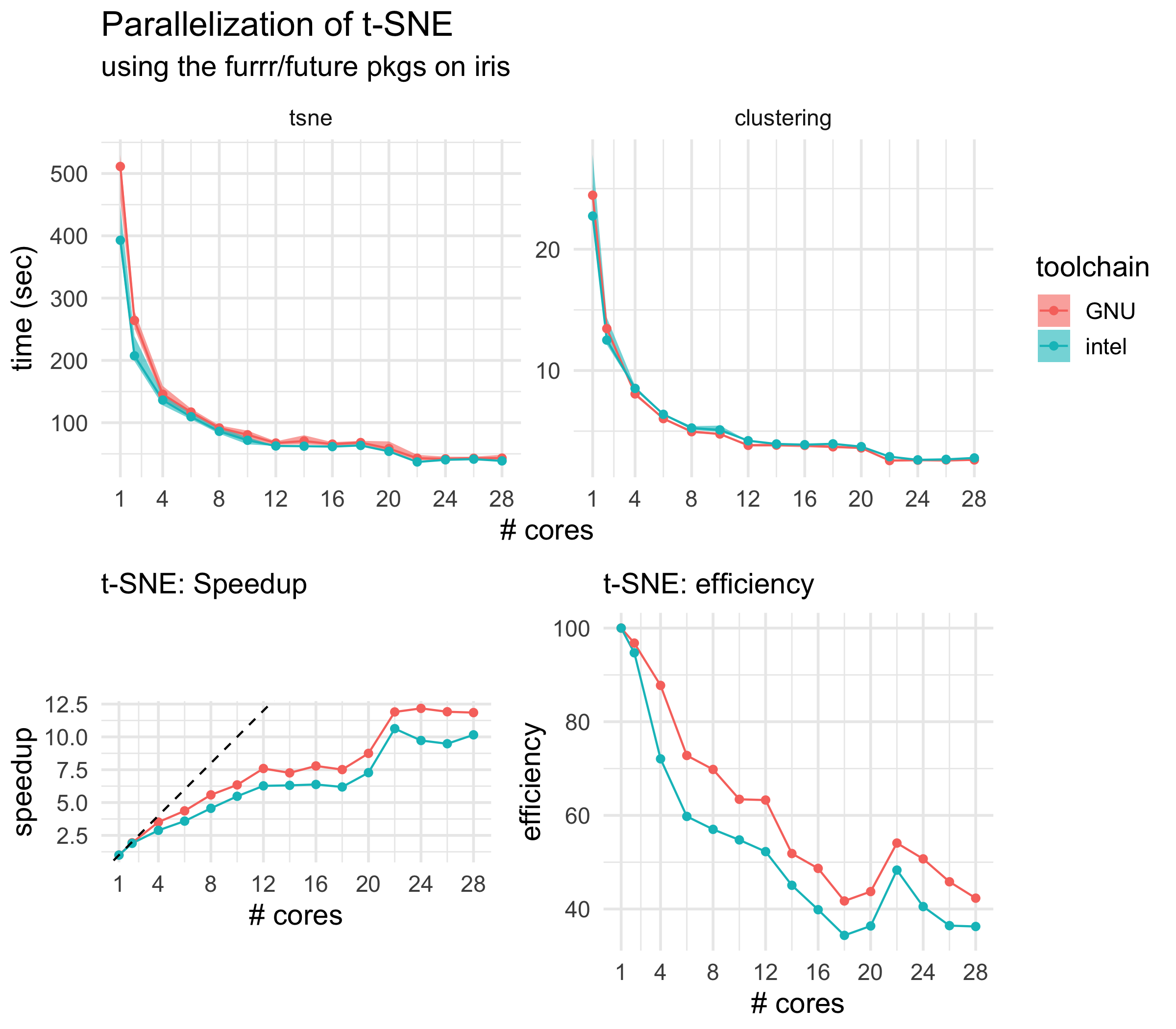#### Animation

just for the fun of it, using the gganimate package, we can visualise how the t-SNE evolves with increasing perplexities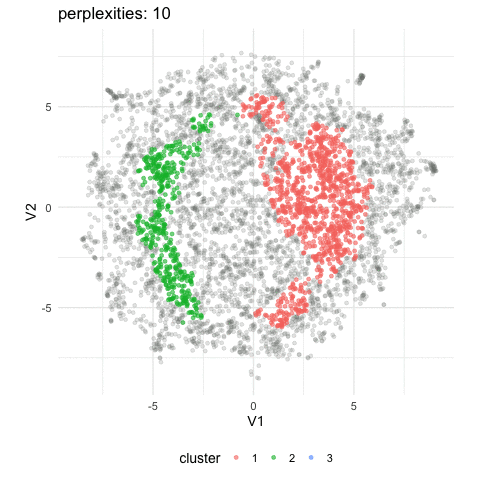If of interest, the code it as follows (install required for gganimate, gifski):

Beware, the animating takes \~ 4 minutes to complete.

library(gganimate)

res %>%
rename(cdbl = c) %>%
filter(perplexities %in% seq(10, 110, 20)) %>%
unnest(tsne) %>%
select(-output) %>%
group_by(perplexities) %>%
mutate(n = row_number()) -> tib_light

tictoc::tic("tweening")
tib_light %>%
ggplot(aes(x = V1, y = V2, group = n, colour = factor(c))) +
geom_point(data = function(x) filter(x, c == 0), colour = "grey50", alpha = 0.2) +
geom_point(data = function(x) filter(x, c != 0), alpha = 0.6) +
transition_states(perplexities, transition_length = 5, state_length = 1) +
ease_aes('cubic-in-out') +
labs(title = "perplexities: {closest_state}") +
labs(colour = "cluster") +
coord_equal() +
theme_minimal(14) +
theme(legend.position = "bottom") -> tib_anim
tictoc::toc()
tictoc::tic("animate")
tib_gif <- animate(tib_anim, nframes = 200, fps = 10)
tictoc::toc()
tib_gif
anim_save("tsne_200.gif", tib_gif)


For the t-SNE example, we cheated a bit by loading a R compressed object I prepared in advance (.rds). It was convenient because loading was fast and the columns and numbers were correctly read.

In real life though, most dataset come as text files with some delimiters. Now we can load the equivalent of the t-SNE data as a .csv file and benchmarks the main tools for this step.

• read.csv() is the base function. Turns out to be slow for large files.
• fread() is the fantastic and fast import function from data.table
• read_csv() is the tidyverse way of reading a csv. Fast than base, slower than data.table.
• vroom() is the recent package that could be the next standard for reading files in the tidyverse dialect. Check the benchmarks for a detailed comparison. It uses the ALTREP introduced in R 3.5.0 that allows to delay actual reading data, making it super fast. Materialization of data comes when you need the exact column and rows.

Here we will benchmark reading only numbers since it is a matrix of doubles.

In your session on iris, in the R console,

• first install required packages
install.packages(c("vroom", "ggplot2", "cowplot", "data.table", "readr", "bench", "ggbeeswarm"), Ncpus = 4)


paste the following code:

library(dplyr)
library(ggplot2)

# must set thread by hand since parallel::detectCores() will detect 28.
# must be 4

tSNEdata_location <- "tSNEdata.csv"
dat_vroom = vroom::vroom(tSNEdata_location, col_types = cols(), progress = FALSE),
dat_vroom_altrep = vroom::vroom(tSNEdata_location, altrep_opts = TRUE, col_types = cols(), progress = FALSE),
check = FALSE, iterations = 50) -> m
# show tables, absolute and relative
m
summary(m, relative = TRUE)
# create a plot of both speed and memory allocations, align the vertical lines
p <- cowplot::plot_grid(autoplot(m),
m %>%
tidyr::unnest() %>%
filter(gc == "none") %>%
mutate(expression = as.character(expression)) %>%
ggplot(aes(x = mem_alloc, y = time, color = expression)) +
geom_point() +
theme_minimal(14), ncol = 1, align = "v")
# save as pdf
ggsave("csv_benchmarks.pdf", p, width = 8)

# A tibble: 5 x 13
expression           min  median itr/sec mem_alloc gc/sec n_itr  n_gc
<bch:expr>       <bch:t> <bch:t>     <dbl> <bch:byt>    <dbl> <int> <dbl>
1 base             205.7ms 210.1ms      4.70        0B    2.35     34    17
2 readr            171.7ms 172.9ms      5.77        0B    0.641    45     5
3 data.table        80.9ms  86.3ms     11.6         0B    1.01     46     4
4 dat_vroom         61.8ms  65.3ms     14.3         0B    1.25     46     4
5 dat_vroom_altrep  42.7ms  47.5ms     21.2         0B    1.84     46     4
# … with 5 more variables: total_time <bch:tm>, result <list>, memory <list>,
#   time <list>, gc <list>

# A tibble: 5 x 13
expression         min median itr/sec mem_alloc gc/sec n_itr  n_gc
<bch:expr>       <dbl>  <dbl>     <dbl>     <dbl>    <dbl> <int> <dbl>
1 base              4.82   4.43      1          NaN     3.67    34    17
2 readr             4.03   3.64      1.23       NaN     1       45     5
3 data.table        1.90   1.82      2.46       NaN     1.57    46     4
4 dat_vroom         1.45   1.38      3.05       NaN     1.94    46     4
5 dat_vroom_altrep  1      1         4.51       NaN     2.88    46     4
# … with 5 more variables: total_time <bch:tm>, result <list>, memory <list>,
#   time <list>, gc <list>vroom is multi-threaded and will use the 4 cores and performed similarly as data.table which was designed to be efficient for numeric data, see the benchmarks done by the author Jim Hester (also maintainer of readr).

### Enclosed R packages environment

this package is still under development but is the promising replacement for packrat. Here is some instructions extracted from the overview vignette

• installation
install.packages("renv")